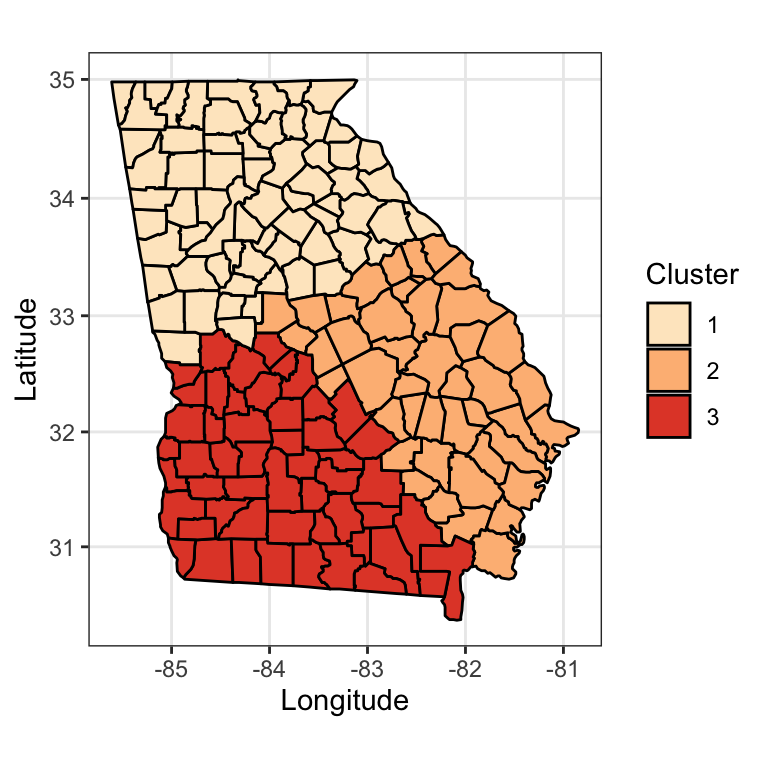This webpage is created as an online supplemental material for the manuscript Heterogeneous Regression Models for Clusters of Spatial Dependent Data.

We consider the second alternative approach as an illustration as the null, no-variation case is trivial, and comparison of the truth and clustering results under the first random true cluster setting can be hard to read on plots. Using the spatial structure of Georgia state, we first partition the map, and create a set of observed $$\mathbf{Y}$$ and covariate matrix $$\mathbf{X}$$. The matrix of great circle distances is provided in a separate GAcentroidgcs.rds file. Note that entries in this matrix have been normalized to have a maximum value of 10. The latitude and longitude of county centroids are provided in GAcentroids.rds. We load both into R workspace first.

distMat <- readRDS("./GAcentroidgcd.rds")
N <- S <- 159For the setting in the figure above, we next define the parameters for each location. For ease of exposition we use Setting 3, which has the strongest signals among all three settings.

## to generate the true clustering setting
asm <- c()
for (i in 1:nrow(centroids)) {
if (centroids$x[i] - 2 * centroids$y[i] < -150) {
asm[i] <- 1
} else if (centroids$x[i] + centroids$y[i] > -51) {
asm[i] <- 2
} else {
asm[i] <- 3
}
}
betaMat <- t(matrix(nrow = 159, ncol = 6, byrow = TRUE))

for (i in 1:159) {
## cluster 1
betaMat[,asm == 1] <- c(9, 0, -4, 0, 2, 5)
## cluster 2
betaMat[,asm == 2] <- c(1, 7, 3, 6, 0, -1)
## cluster 3
betaMat[,asm == 3] <- c(2, 0, 6, 1, 7, 0)
}

Next, we generate the covariates i.i.d. from the standard normal distribution, and generate the spatial random effect $$\textbf{W}$$ from the multivariate normal distribution with zero mean, and $$\exp(-\mbox{GCD}/4)$$ covariance matrix.

set.seed(3)

X <- matrix(rnorm(159 * 6), nrow = 159)
W <- MASS::mvrnorm(1, mu = rep(0, 159), Sigma = exp(-distMat / 4))
Y <- diag(X %*% betaMat) + W + rnorm(159)

As described in the main text, the nimblefunction() is defined.

library(nimble)
SLMMCode <- nimbleCode({
for (i in 1:S) {
y[i] ~ dnorm(mu_y[i], tau = tau_y)
mu_y[i] <- b[i, 1] * x1[i] + b[i, 2] * x2[i] +
b[i, 3] * x3[i] + b[i, 4] * x4[i] + b[i, 5] * x5[i] +
b[i, 6] * x6[i] + W[i]

b[i, 1:6] <- bm[latent[i], 1:6]

latent[i] ~ dcat(zlatent[1:M])
}
tau_y ~ dgamma(1, 1)

for (j in 1:S) {
for (k in 1:S) {
H[j, k] <- exp(-Dist[j, k]/phi)
}
}

W[1:S] ~ dmnorm(mu_w[1:S], prec = prec_W[1:S, 1:S])
prec_W[1:S, 1:S] <- tau_w * inverse(H[1:S, 1:S])

phi ~ dunif(0, D)
tau_w ~ dgamma(1, 1)

mu_w[1:S] <- rep(0, S)

for (k in 1:M) {
bm[k, 1:6] ~ dmnorm(mu_bm[1:6], cov = var_bm[1:6, 1:6])
}
var_bm[1:6, 1:6] <- 1/tau_bm * diag(rep(1, 6))
tau_bm ~ dgamma(1, 1)

for (j in 1:6) {
mu_bm[j] ~ dnorm(0, 1)
}

zlatent[1:M] <- stick_breaking(vlatent[1:(M - 1)])

for (j in 1:(M - 1)) {
vlatent[j] ~ dbeta(1, alpha)
}

alpha ~ dgamma(1, 1)

})

The list of data, constant parameters, as well as starting values, are declared.

SLMMdata <- list(y = Y, x1 = X[,1], x2 = X[,2], x3 = X[,3],
x4 = X[,4], x5 = X[,5], x6 = X[,6],
Dist = distMat)

SLMMConsts <- list(S = 159, M = 50, D = 100)
SLMMInits <- list(tau_y = 1,
latent = rep(1, SLMMConsts$S), alpha = 2, tau_bm = 1, mu_bm = rnorm(6), phi = 1, tau_w = 1, vlatent = rbeta(SLMMConsts$M - 1, 1, 1)
)

We invoke MCMC to get the results. The total number of iterations is set to be 50000 with thinning interval 10.

mcmc.out <- nimbleMCMC(code = SLMMCode, data = SLMMdata,
constants = SLMMConsts,
inits = SLMMInits,
monitors = c("bm","b","phi", "tau_w",
"alpha", "latent", "tau_y"),
niter = 50000,
thin = 10, nchains = 1, setSeed = TRUE)
## NAs were detected in model variables: bm, logProb_bm, W, logProb_W, b, mu_y, logProb_y.
## |-------------|-------------|-------------|-------------|
## |-------------------------------------------------------|
## discard the first 2000 burn-in samples
library(coda)
pos_mcmc <- as.mcmc(mcmc.out[-c(1:2000),])

The next step is to take out the 159-dimensional latent cluster congifuration vector $$\mathcal{Z}$$, and obtain the membership matrix as in Equation (12). Based on the list of 3000 membership matrices, the empirical probability matrix is calculated, and the iteration that has the closest sum of squared distance from $$\overline{B}$$ is found.

latentZMat <- pos_mcmc[, 1256:1414]

membershipList <- purrr::map(1:nrow(latentZMat), .f = function(x) {
outer(latentZMat[x,], latentZMat[x, ], "==")
})

## the empirical probability matrix
bBar <- Reduce("+", membershipList) / length(membershipList)

## sum of squared differences
lsDist <- purrr::map_dbl(membershipList, ~sum((.x - bBar) ^ 2))

## find the optimal iteration, and take as the final inferenced result
## if there are multiple optimal iterations, take the first one
mcluster <- which.min(lsDist)
finalCluster <- as.numeric(latentZMat[mcluster,])

The Rand index is calculated:

RI <- fossil::rand.index(finalCluster, asm)
RI
##  0.8490566

The clustered results visualized together with the original partition: